# Bash Scripting – Until Loop

• Difficulty Level : Medium
• Last Updated : 18 Jan, 2022

The Bash has three types of looping constructs namely for, while, and until. The Until loop is used to iterate over a block of commands until the required condition is false.

Syntax:

```until [ condition ];
do
block-of-statements
done```

Here, the flow of the above syntax will be –

• Checks the condition.
• if the condition is false, then executes the statements and goes back to step 1.
• If the condition is true, then the program control moves to the next command in the script.

### Example:

```#!/bin/bash
echo "until loop"
i=10
until [ \$i == 1 ]
do
echo "\$i is not equal to 1";
i=\$((i-1))
done
echo "i value is \$i"
echo "loop terminated"```

Output: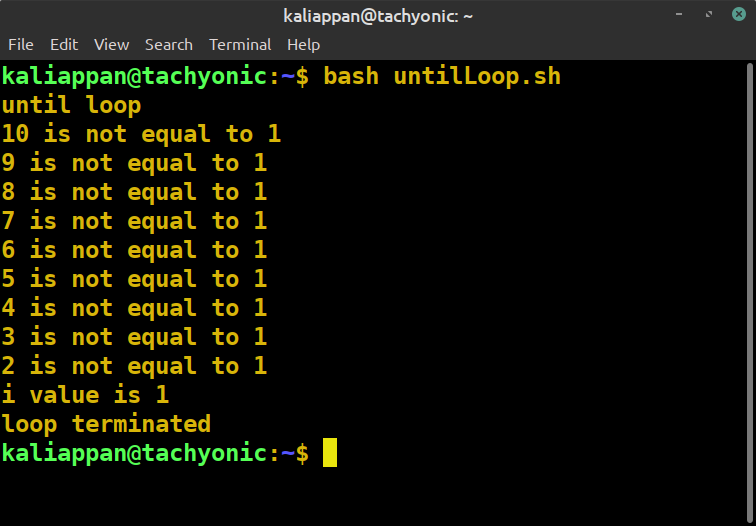## Infinite Loop using Until

In this example the until loop is infinite i.e it runs endlessly. If the condition is set in until the loop is always false then,  the loop becomes infinite.

### Program:

```#!/bin/bash
## This is an infinite loop as the condition is set false.
condition=false
iteration_no=0
until \$condition
do
echo "Iteration no : \$iteration_no"
((iteration_no++))
sleep 1
done```

Output: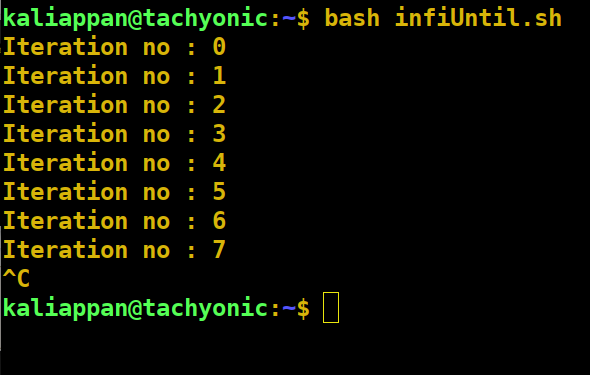## Until Loop with break and continue

This example uses the break and the continue statements to alter the flow of the loop.

• break: This statement terminates the current loop and passes the program control to the following commands.
• continue: This statement ends the current iteration of the loop, skipping all the remaining commands below it and starting the next iteration.

### Program

```#!/bin/bash
## In this program, the value of count is incremented continuously.
## If the value of count is equal to 25 then, the loop breaks
## If the value of count is a multiple of 5 then, the program
## control moves to the next iteration without executing
## the following commands.
count=1

# this is an infinite loop
until false
do

if [[ \$count -eq 25 ]]
then

##   terminates the loop.
break
elif [[ \$count%5 -eq 0 ]]
then

## terminates the current iteration.
continue
fi
echo "\$count"
((count++))
done```

Output: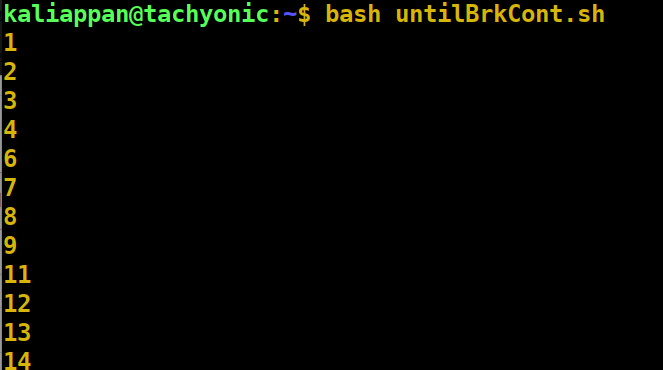## Until Loop with Single condition

This is an example of an until loop that checks for only one condition.

### Program

```#!/bin/bash
# This program increments the value of
# i until it is not equal to 5
i=0
until [[ \$i -eq 5 ]]
do
echo "\$i"
((i++))
done```

Output: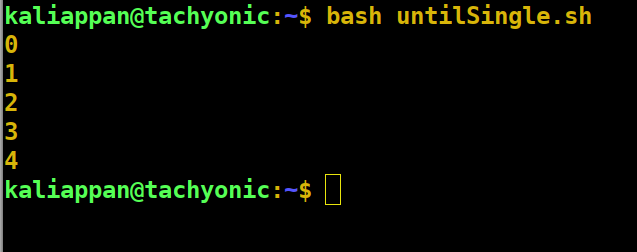## Until Loop with Multiple conditions

Until loop can be used with multiple conditions. We can use the and : ‘&&’ operator and or: ‘||’ operator to use multiple conditions.

### Program

```#!/bin/bash
##  This program increments the value of
## n until the sum is less than 20
##   or the value of n is less than 15
n=1
sum=0
until [[ \$n -gt 15  || \$sum -gt 20 ]]
do
sum=\$((\$sum + \$n))
echo "n = \$n & sum of first n = \$sum"
((n++))
done```

### Output: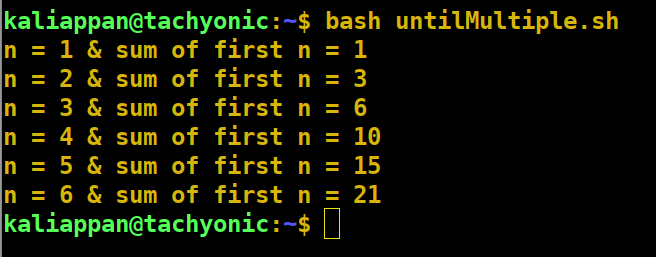## Exit status of a command:

We know every command in the shell returns an exit status. The exit status of 0 shows successful execution while a non-zero value shows the failure of execution. The until loop executes till the condition returns a non-zero exit status.

My Personal Notes arrow_drop_up